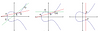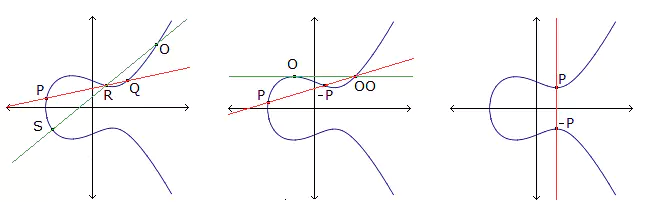# Transitive A6-invariant k-arcs in PG(2, q)

### Journal article

M. Giulietti, G. Korchmáros, S. Marcugini, F. Pambianco
Des. Codes Cryptogr., 2011

Semantic Scholar ArXiv DBLP DOI
Cite

### Cite

APA
Giulietti, M., Korchmáros, G., Marcugini, S., & Pambianco, F. (2011). Transitive A6-invariant k-arcs in PG(2, q). Des. Codes Cryptogr.

Chicago/Turabian
Giulietti, M., G. Korchmáros, S. Marcugini, and F. Pambianco. “Transitive A6-Invariant k-Arcs in PG(2, q).” Des. Codes Cryptogr. (2011).

MLA
Giulietti, M., et al. “Transitive A6-Invariant k-Arcs in PG(2, q).” Des. Codes Cryptogr., 2011.

## Abstract

For q = pr with a prime p ≥ 7 such that $${q \equiv 1}$$ or 19 (mod 30), the desarguesian projective plane PG(2, q) of order q has a unique conjugacy class of projectivity groups isomorphic to the alternating group A6 of degree 6. For a projectivity group $${\Gamma \cong A_6}$$ of PG(2, q), we investigate the geometric properties of the (unique) Γ-orbit $${\mathcal{O}}$$ of size 90 such that the 1-point stabilizer of Γ in its action on $${\mathcal O}$$ is a cyclic group of order 4. Here $${\mathcal O}$$ lies either in PG(2, q) or in PG(2, q2) according as 3 is a square or a non-square element in GF(q). We show that if q ≥ 349 and q ≠ 421, then $${\mathcal O}$$ is a 90-arc, which turns out to be complete for q = 349, 409, 529, 601,661. Interestingly, $${\mathcal O}$$ is the smallest known complete arc in PG(2,601) and in PG(2,661). Computations are carried out by MAGMA.

Share